﻿ A Possible Electrochemical Route to a Thermodynamic Redox Reaction Equilibrium Constant in Secondary Education: An Attempt to Come from Science Fiction to Science Education?Publications are Open
Access in this journal
Article Versions
Export Article
• Normal Style
• MLA Style
• APA Style
• Chicago Style
Research Article
Open Access Peer-reviewed

### A Possible Electrochemical Route to a Thermodynamic Redox Reaction Equilibrium Constant in Secondary Education: An Attempt to Come from Science Fiction to Science Education?

World Journal of Chemical Education. 2020, 8(3), 128-140. DOI: 10.12691/wjce-8-3-5
Received June 19, 2020; Revised July 20, 2020; Accepted July 30, 2020

### Abstract

A description is given of an electrochemistry refresher course as a basis for tabulated standard electrode potentials using the Nernst equation and relating chemical equilibrium constants. In connection with their professional experiences the participating teachers carried out measurements of the voltage of a self-built electrochemical cell as described in a final examination task. In this task students had to calculate the voltage using tabulated E0 values. The sign of the measured and calculated voltage appeared to be different. Measurement of current-potential curves of different cells with the help of a Poggendorf compensation circuit affirmed the surmise of a thermodynamic context rather than an empirical one for the Nernst equation: reversible reactions have to be distinguished from spontaneous ones. Even the best measuring cells did not give a good correspondence simultaneously for E0 and the factor RT/nF in the Nernst equation, which was not only due to insufficient time to get equilibrium results. This led to the use of cells taken from literature and the concept of activity coefficient calculated from the Debije-Hückel theory. So generalisation (the mathematical form of the Nernst equation), idealisation (very diluted solution), modelling (corpuscularity) and specification (calculation of the activity coefficient) are necessary for a correct interpretation of apparent empirical values like a standard electrode potential or a chemical equilibrium constant.

### 1. Introduction

Science is not just an opinion, it is a complex collection of facts, held together by models and theories. Some of these facts, like ‘wood can be burnt’, are obvious and connectible to everyone’s experience, but others, like the value of the sun’s mass or the meaning of electronic orbitals, are more difficult to agree on. A correct interpretation of the facts presented in scientific handbooks and tables demands a long learning path. Sometimes, even scientifically trained people miss a good understanding, which may be illustrated with the following example. A group of pre university chemistry teachers attended a refresher course on electrochemistry and were surprised when they measured the potential difference of the following galvanic cell: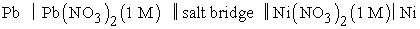In 1981 this cell was presented in a task in the Dutch final chemistry examination and students had to calculate its potential difference on the basis of the values of the standard electrode potentials in BINAS 1, a table book, which students have to use during the final examinations (see also supplementary material 1). According to the tabulated values the lead electrode would be the positive one, but in practice the teachers measured the opposite: the nickel electrode turned out to be the positive one. Hitherto, the standard electrode potentials and the Nernst equation to calculate cell potential differences, seemed unproblematic facts and using these in this way was seen as a normal procedure. Now they became the starting point for an investigation into their significance. Obviously, the tabulated standard electrode potentials could not be measured directly from this cell.

### 2. Learning and Teaching Problems with Electrochemistry

In the 1980s the Department of chemical education of Utrecht University in The Netherlands decided to offer a refresher course on electrochemistry (see supplementary material 2). Herein the Department was led by national and international literature on the interrelated problems with chemical equilibrium, electrochemistry and thermodynamics, and on the basis of its own experiences with these difficulties. Already in 1982 Bojczuk reported on this matter for the United Kingdom 2, De Jong for The Netherlands 3, Finley, Stewart and Yarroch for the USA 4 and in 1987 Butts and Smith for Australia 5. The great complexity of the topic of electrochemistry for students as well as for teachers is shown by the still growing literature 6, 7, 8, 9. Related to electrochemistry are problems in chemical equilibrium 10, 11, 12, 13, 14 and thermodynamics 15, 16, 17, 18, 19, 20.

Reasons for these problems can be found in the abstract content in these topics 21, 22, 23, the persistence of non-scientific views 13, 14, or even in problematic passages in college books 24.

In our Department a clear relation was seen to contemporary research dealing with an empirical route to a qualitative concept of chemical equilibrium by means of visible and reversible reactions in secondary education 25 and learning to measure in tertiary education 26. In our view special attention should be paid to measurement of electrode potentials, and a possible use of the Nernst equation for an empirical foundation of a chemical equilibrium constant.

### 3. Learning to Measure Cell Potential Differences

The attendants to the refresher course on electrochemistry constructed a variety of electric cells and measured their cell potential differences in various ways (see supplementary material 3). The cell potential values as measured and calculated from BINAS, are reproduced in Table 1 and were discussed with all participants.

The differences between measured and calculated values are mostly reasonable. Two exceptions are remarkable:

- the measured potential difference in cell 4

(Pb│PbSO4 (s) │Fe2+ (0.1 M), Fe3+ (0.1 M) │Pt) is significantly lower than the calculated value;

- the sign difference between the measured and the calculated value of the central examination cell (1, 2).

Not only did the well-known formula for Nernst’s law become problematic, but the route from measurements of potential difference of real systems towards the tabulated calculated values in the handbooks as well. A cognitive conflict arose when the teachers discovered that the BINAS values and the Nernst-equation are not empirical. The question arose if they are thermodynamic ones. On the basis of their own measurements a need was created for the meaning of tabulated standard electrode potentials and equilibrium constants like solubility products, acid-base constants and complex dissociation constants in the information book BINAS. Because of the differences between the results of the teachers the quality of the experiments had to be enhanced too.

### 4. Thermodynamic Reversibility of Chemical Reactions

During the refresher course the attendants had to use the Poggendorf compensation circuit in order to have the chemical reaction in an electric cell to go backwards (electrolysis cell = electrochemical reactor) and forwards (galvanic cell = electric energy source) by having the current going either direction. Equilibrium exists in the situation of no current. The number of measuring pairs of the potential and current are dependent of the time available for adjustment of the cell in order to get constant and reproducible readings.

In these experiments experience can be gained with the reversibility of the electrode reactions in thermodynamic view (see Figure 1 and Figure 2).

• Figure 1. Cell with thermodynamic reversible electrode reactions (Measurements on the cell: Zn │ZnCl2 (1 M) ║ KCl (1 M) ║CuCl2 (1 M)│Cu)
• Figure 2. Cell with thermodynamic non-reversible electrode reactions (Measurements on the cell: Pb│Pb(NO3)2 (1 M) ║ KNO3 (1M) ║Ni(NO3)2 (1 M)| Ni)

The reaction in both cells can be called reversible in a kinetic sense. However, in a thermodynamic sense only the reaction shown in Figure 1 can be called reversible and that in Figure 2 thermodynamic irreversible. To arrive at an equilibrium constant with the aid of the Nernst equation, measurements like those shown in Figure 2 point to an improvement of the experiments by:

- control of thermodynamic reversibility, reaching equilibrium

- choice of one ideal, polarisable electrode of interest with a thermodynamic reversible half-reaction and one non-polarisable reference electrode like a calomel electrode or Ag/AgCl electrode 27. Thereby a diffusion potential is avoided (see supplementary material 4).

### 5. Checking the Nernst-equation as an Empirical Law with the Help of a Better Cell Arrangement

In Dutch secondary education the Nernst equation is described as an empirical law, stating that at 293 K the electrode potential E corresponding to the half-reaction Red ⇌ Ox + n e- equals the sum of the tabulated standard electrode potential E0 and the logarithm of the molarities of the species participating in the half-reaction in question: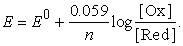(1a)

In order to validate this relation each group of participants in the refresher course constructed a cell with the electrode and electrolyte of interest together with a reference electrode with a constant potential (see Table 2).

In general, the measuring results were very diverse. Straight lines (estimated or determined with a linear regression program) were found in ten cases, less than half the number of measurements. Stirring or, for lower concentrations, addition of a carrier electrolyte like KNO3 did not bring about any improvement. In one measurement, correspondence was reached between the E0-value measured and the tabulated one (Pb/Pb2+). Correspondence between a measured slope and those calculated from the Nernst equation (1a) was found four times for Pt │Fe2+ (x M) Fe3+ ((0.2 - x) M) and once for Cu│Cu2+ (x M).

Examples of good correspondence with one of the factors in the Nernst equation are shown below (Figure 3 and Figure 4).

• Figure 3. Good correspondence between cell potential and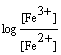from the Nernst equation
• Figure 4 Good correspondence with the Nernst equation in relation to the E0-value

The E0 of the Ag/AgCl electrode is 0.222 V and the E0 of the redox couple Fe3+/Fe2+ = 0.77V. On the basis of equation (1a) the potential difference of the cell can be calculated as: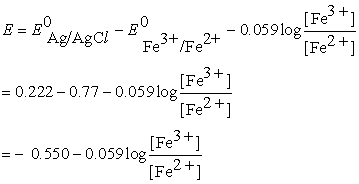The slope of the graph is in reasonable accordance with the theoretical one, but the E0 value for the redox couple Fe3+/Fe2+ deduced from this result is not.

The E0 of the calomel electrode is 0.248 V, so the Nernst equation (1a) for this cell will be: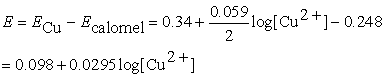The slope is less than half the theoretical one, but the ‘E0’ value is in a better range than the one in Figure 3.

Probable causes for these bad results are:

- the context of this course (and school!) is not appropriate for this kind of measurements. To reach an equilibrium situation one needs more time, sometimes one day for one measuring point. Therefore, the step was taken towards literature cells later on because those values were good ones to calculate.

- the physical state of the electrodes: very pure chemical metals, especially the hard ones, display tensions wich influence the electrode potential. Hence, accurate, reproducible measurements are executed with the help of amalgams, not appropriate for a secondary school for reasons of safety.

From the results obtained in the first two meetings of the refresher course, we had to suppose that the tabulated E0values and the Nernst equation have a thermodynamic and not an empirical character.{1} Also, the basis for a cognitive conflict was laid to ensure a conceptual change 28.

### 6. Some Relevant Thermodynamic Topics on Equilibrium Description

First of all, phenomenological thermodynamics deals with reversible processes, i.e. a change that can be reversed by an infinitesimal modification of a variable. A reversible process goes from the initial state to the final state through an infinite series of equilibrium states 29, 30. As a consequence of this idealisation a “spontaneous” chemical reaction is irreversible. Secondly, the description of a reaction of perfect gases and accompanying equilibrium constant is given by the van‘t Hoff reaction isotherm: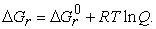In the reaction quotient Q each pressure is divided by a standard pressure, for logarithms can only be taken from a number. At equilibrium ΔGr = 0 and Q = K, the equilibrium constant in terms of (relative) equilibrium pressures of the participating gases.

For the description of reactions in other situations (non-ideal gases, non-electrolyte solutions, electrolyte solutions) the mathematical form of the above equation is maintained. But in order to bring these descriptions in correspondence with the experimental results of enthalpy and entropy determination (ΔG = ΔH -TΔS) another substance specific quantity for composition is chosen: for gases fugacity, being the relative partial pressure provided with a correction factor, the fugacity coefficient; for the condensed phase a quantity called activity is used, mostly relative molality, corrected by the so called activity coefficient γ.

K (now presented as Ka) can be split up into Km, a quotient of relative molalities and Kγ, a quotient of substance specific correction factors, leading to Ka = Km. Kγ. This is the thermodynamic equilibrium constant expressed in activities.

For an electrochemical cell reaction the van ‘t Hoff reaction isotherm,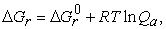can be transformed into the Nernst equation with the help of the relation: ΔGr = - n F Δ E: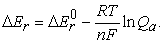At equilibrium Δ E = 0, hence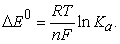(1b)

This formulation has general validity, contrary to equation (1a) given in Dutch secondary school books. The Nernst equation and the tabulated values of standard electrode potentials have a thermodynamic and not an empirical character.

Nevertheless, potential measurement appears easier to do and has a better chance of success than measurement of enthalpy and entropy, although there must be a solution for the activity coefficients, either by measurement or by calculation.

### 7. The Concept of Activity and the Limiting Law of Debye-Hückel for Electrolyte Solutions

The attendants to the refresher course had to learn how tabulated E0 values can be found by measuring results as the start. Therefore three steps were taken:

a. results of equilibrium measurements were selected from reversible cells from literature;

b. molality was chosen as a measure of composition because of its independence of temperature, unlike from molarity;

c. each molality value was corrected with a product factor specifically belonging to it, the activity coefficient γ. The product γ∙ m is called activity a and can be found by measuring e.g. the osmotic pressure value or the vapour pressure of a solution.

The activity coefficient expresses the interaction between the particles in the solution. For very diluted solutions: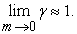The mathematical form of the composition fraction and other expressions dealing with the properties of the solution were maintained (a form of generalisation).

An electrolyte solution is always neutral, so positive and negative ions must be added to or extracted from the solution together. Because all ions contribute to the value of the activity, the separate ion activities (a+ = γ+m+ and a- = γ- m-) cannot be measured, because one ion molality cannot be varied independently from that of other ions. Also, the separate ion activities are hypothetical. Hence, electrolyte solutions are described with mean activity coefficients (γ±) and these values can be calculated on the basis of measurements.

For an important part, electrostatic interaction between ions determines the properties of an electrolyte solution and hence the value of the mean activity coefficient γ±. For the description of the behaviour of mixtures of electrolyte solutions Lewis introduced another kind of measure of concentration that takes into account the magnitude of the charge of the ions, the ionic strength:(2)

zi being the charge of ionic species i with molality mi.

Empirically, he found that in diluted solutions the activity coefficient of a strong electrolyte is the same in all solutions of the same ionic strength. Debye and Hückel gave a theoretical foundation to the Lewis rule for very diluted electrolyte solutions. Their limiting law runs as follows: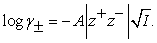(3)

The factor A contains no reference to the electrolyte, but only to the solvent, so that the Lewis rule is proved in this approximation.

In this contribution we confine ourselves to electrolytes with only univalent anions and cations. (A general derivation is found in the supplementary material 5.) The following cells were selected from literature 31, 32 and used during the refresher course:

i) (Pt) H2 │HCl (m)│Hg2Cl2(s) │Hg

ii) (Pt) H2 │HCl (m)│AgCl(s) │Ag

With the accompanying reaction equations:

i) H2 + Hg2Cl2 ⇌ 2 H+ + 2 Hg + 2 Cl-

ii) H2 + 2 AgCl ⇌ 2 H+ + 2 Ag + 2 Cl-

For these cells found in literature the combination of equation (2) and (3) yields: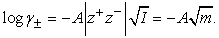(4)

### 8. Relation between E, γ± and m for Univalent Ions

At first, an expression for the Nernst equation of the solution of a strong electrolyte must be found with the help of definitions of the mean activity and mean activity coefficient.

Let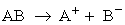be the chemical equation for the dissolution of the strong univalent electrolyte AB.

The solute activity a is defined as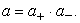(5)

The mean activity a± is defined as the geometrical mean of the separate ion activities: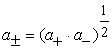(6)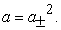(7)

Substituting a+ = γ+m+ and a- = γ- m- in equation (6) leads to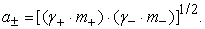(8)

Because m+ = m_ = m we can write: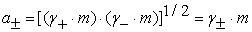(9)

with a definition of the mean activity coefficient analogous to the mean activity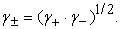(10)

Substitution of equation (8) and (9) in the Nernst equation (1) produces the following result: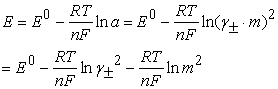(11)

{2}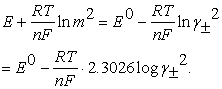(12)

Substituting equation (4) into equation (12) gives: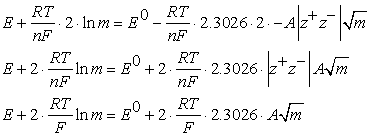(13)

The value of the constant A = 0.509 at 298 K, so the following can be arrived at: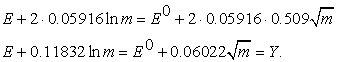(14)

The essential leap towards the solution of finding the value of E0 is splitting up the Nernst equation into the parts that are shown in equation (14). The factors on the left hand are known as the molality and as the measuring result of the molality used. The factors on the right hand can be found by plotting those on the left hand against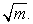A diagram of Y versus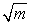results, by extrapolation to infinite dilution (m = 0), in a tabulated value E0, which is, by its very nature, a value within a thermodynamic and corpuscular context according to the interpretation of activity coefficients presented above.

### 9. Determination of a Standard Electrode Potential by Splitting up the Nernst Equation

Next we tried to determine for these cells by calculation:

- the validity of the linear relation between E and log m;

- the value of E0 and the value of γ± for some concentrations.

For convenience, equation (14) can be written as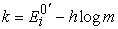with the subscript i referring to the number of the cell under consideration and: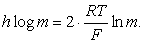Also:With these values the results in Table 3 can be arrived at:

• Figure 5. Comparison of γ± computed in the course and from literature for cells i and ii (▲: values from Hills and Ives (1951), ●: calculated values cell I, ■: calculated values cell ii)

The final conclusion is inescapable: the Nernst equation is not an empirical law and contains no empirical standard electrode potential. Also, a direct calculation of equilibrium constants of redox reactions as done in the final examination task, does not result in empirical values, in spite of the best equilibrium measurements with the best electrolyte cell at hand.

Table 3 and Figure 5 show that the simple version of the limiting law of Debye-Hückel produces reasonable results for E0 and γ±, certainly in the context of this refresher course. It is necessary to use the model of the limiting law because:

1. Ebullioscopic measurements at descending concentrations give a straight line through the origin of the diagram; in the case of potential differences measurements this is not the case as the resulting line intersects the y-axis at the E0 value to be measured.

2. Without any objection measuring at low concentration has two disadvantages:

a. There is no guarantee that further dilution has no effect on γ± as lim γ± = 1 applies to m = 0, but in real situations m > 0.

b. Measurements of very diluted solutions are not the best possible ones, and therefore, a straight line only through these points is not the most accurate one.

### 10. Discussion and Evaluation

10.1. Chemical and Pedagogical Quality of the Course

The choice for a Poggendorf circuit in combination with a measurement of E vs. I - curves appeared an immediate success: within a large number of hardly interpretable curves a limited number of symmetrical curves with reference to the V -axis (linear or sigmoidal) were found. They represent an extension of the equilibrium concept, as not only the reactions are reversible, but the accompanying changes in energy as well. This means we deal with an reversible, equilibrium reaction in a thermodynamic sense. Because spontaneous reactions are not reversible in a thermodynamic sense, at I = 0 we have an opportunity to measure the equilibrium potential difference and find the relation between E0 and K.

To investigate the relation between cell potential difference and particle concentration further, either a concentration cell with a Pb/PbSO4 electrode or a cell with a reference electrode with constant potential (Ag/AgCl or calomel electrode) was made. Some of the results showed straight lines, but either the slope produced corresponded to the Nernst equation or the intersection with the E axis agreed with the tabulated E0 value of the half-reaction of interest. Never these two aspects did appear simultaneously. The conclusion that the Nernst equation, the tabulated E0 values and the equilibrium constants calculated from these are not empirical, gained more confidence.

Reasons for these bad results can be found in the lack of time to reach equilibrium, and in the fact that many metal electrodes display tension involving different potentials. As a consequence, for closer examination we used good equilibrium measurements from literature.

By introducing the mean activity a± and the mean activity coefficient γ±, calculating the last one as a function of m for very dilute electrolyte solutions with the help of the corpuscular limiting law of Debye-Hückel, the Nernst equation can be split up into a measuring part (E, m) and a calculating part (E0, γ±). With this molality-dependent activity coefficient the attendants got E0 results in very good agreement with the literature. The route to a chemical equilibrium constant via the Nernst equation proved realizable, in spite of the specific electrochemical problems of charge and electrodics. The connection between the experiences of the teachers and their motivation for the successive tasks appeared to be strong. Their appreciation was great and there was scarcely any absenteeism.

10.2. Electrode Kinetics and Catalysis

Until the second half of the twentieth century the main attention about the current/potential curves in electrochemistry was directed at thermodynamics: the relation between charge and converted quantity of matter (Faraday), ionics (Debye-Hückel) and the double layer theory (Gouy-Chapman). Electrodics, the study of electrode kinetics and - catalysis became more important after the so-called polarography with interest in metal reductions on a dropping mercury electrode with diffusion processes under control. For important industrial processes, like electrolysis and the production of semiconductors, more attention was needed than merely seeing an electrode as a reservoir for electrons. Electrode architecture and the electrode-electrolyte interface will influence activity and selectivity of the reactions 30. E.g. the rotating disc ring electrode makes measurement on short living intermediates possible. Reduction of nitric acid in an environment of sulfuric acid produces NH3with a copper cathode, and NH2OH with a lead cathode.

10.3. Limit of the Conceptual Structure in Secondary Education

The conceptual structure of the chemistry curriculum in secondary education in the period when the refresher course was given, is described by Van Berkel 34. According to him this structure appeared to be very rigid and a blockade for renewal worldwide. It can be shown that the foundation of the chemical equilibrium constant by means of a kinetic deduction appeared to be questionable, and on the other hand its foundation on the Nernst equation is a purely phenomenological thermodynamic one with accompanying difficulties. Therefore, this topic arrives outside of this chemical conceptual structure. Hence it seems possible that the quantitative equilibrium constant will disappear from the curriculum in time. Moreover, the international trend in development of secondary school chemistry education is rather in the direction of chemistry embedded in a social context, than scientific chemistry. In a personal discourse one of the authors of the BINAS handbook admitted the virtual character of the calculations with the Nernst equation but defended its position in the curriculum as habituation to some quantitative description of equilibrium and the possibility to make predictions.

10.4. Blockading effect of the Dutch Education Act

In The Netherlands the content of the chemistry curriculum is determined primarily by the government (final central examination and table book to be used) and subsequently by commercially available exam-driven textbooks. Experiences of teachers, results of educational research or critical reflection on the content by teachers and researchers in intensive discussion as reported above have not resulted in a change in curriculum content or educational methodology. In The Netherlands, reform of the secondary school chemistry education by quality of teacher courses and educational research is blockaded, on the one hand by the structure of the law and the separation of responsibilities by government driven institutions, and on the other hand by quality-driven institutions like teachers and their educators. Total revision efforts, frequently accompanied by modernisation of content, have a periodicity of some decennia. In The Netherlands, such a revision has taken place recently, so at the moment the chance for a possible reform is very small. This means that students and teachers will still have to be able to deal with equilibrium problems, although they might require a better foundation.

### 11. Retrospect

The trend towards more context-based chemistry opens the possibility for teachers and students to measure on and teach about real systems. Sometimes the outcomes of such measurements can be puzzling, but can also contribute to the sense of how scientists are trying to describe phenomena in such a way that coherence between different regularities becomes clear. We think it is better to have students develop a good, qualitative understanding of complicated chemical concepts like electrochemistry, than have them solve non-realistic problems in a virtual chemical reality in which tables with data serve as a means to get outcomes without the possibility to check if they are sensible. Calculation of molarities on the basis of quasi-empirical cell voltages belongs to science fiction, not to science. Persistence on the trail of answering questions with the help of tables non-fully understood, is a choice, not a necessity.

### Acknowledgements

We wish to thank H.P.M. Bastiaansen for his careful reading of the manuscript and his valuable proposals for improvement.

### Associated Content

1 The final examination task with the description of the lead nickel cell.

2. A brief description of the content and organisation of the refresher course in electrochemistry that formed the basis for this article.

3. Descriptions of the construction of the electrochemical cells that were built and measured, including the voltage apparatus used.

4. Motivation for the cells used in order to arrive at the most reliable cell voltages for computing electrode standard potentials.

5. A general derivation of the equation on the basis of which a sound thermodynamic standard electrode potential can be computed.

### Notes

1. Other thermodynamic values in the BINAS handbook are those of the enthalpy of formation and of combustion, and the molar boiling point elevation and freezing point depression.

2. Besides our choice for a binary mono electrolyte for the easiest possible computation of the activity, in this derivation the following aspects are important: - composition taken in terms of relative composition in order to be able to take the logarithm of numbers without units; - a condensed phase has activity a = 1, and gas phase is under standard conditions (H2: p = 1).

### References

  Beerens, J.H.M., Hafkenscheid, G.A.M., Jansen, A.I., de Lange, A., Stam, C.W. and Verkerk, G., Binas. Wolters-Noordhoff, Groningen, 1977. In article  Bojczuk, M., “Topic difficulties in o- and a-level chemistry”, School Science Review, 63 (224), 545-551, 1982. In article  De Jong, O., “Moeilijke onderwerpen [difficult topics]”, Chemisch Weekblad, 78, 90-91, 1982. In article  Finley, F.N., Stewart, J. and Yarroch, W.L., “Teachers' perceptions of important and difficult science content”, Science Education, 66 (4), 531-538, 1982. In article View Article  Butts, B. and Smith, R., “Hsc chemistry students' understanding of the structure and properties of molecular and ionic compounds”, Research in Science Education, 17 (1), 192-201, 1987. In article View Article  Ahtee, M., Asunta, T. and Palm, H., “Student teachers' problems in teaching 'electrolysis' with a key demonstration”, Chemistry Education Research and Practice, 3 (3), 317-326, 2002. In article View Article  De Jong, O., Acampo, J. and Verdonk, A., “Problems in teaching the topic of redox reactions: Actions and conceptions of chemistry teachers”, Journal of Research in Science Teaching, 32 (10), 1097-1110, 1995. In article View Article  Özkaya, A.R., “Conceptual difficulties experienced by prospective teachers in electrochemistry: Half-cell potential, cell potential, and chemical and electrochemical equilibrium in galvanic cells”, Journal of Chemical Education, 79 (6), 735, 2002. In article View Article  Osman, K. and Lee, T., “Impact of interactive multimedia module with pedagogical agents on students' understanding and motivation in the learning of electrochemistry”, International Journal of Science and Mathematics Education, 12 (2), 395-421, 2014. In article View Article  Cheung, D., “The adverse effects of le châtelier's principle on teacher understanding of chemical equilibrium”, Journal of Chemical Education, 86 (4), 514, 2009. In article View Article  Cheung, D., Ma, H.-j. and Yang, J., “Teachers' misconceptions about the effects of addition of more reactants or products on chemical equilibrium”, International Journal of Science and Mathematics Education, 7 (6), 1111-1133, 2009. In article View Article  Azizoglu, N., Alkan, M. and Geban, Ö., “Undergraduate pre-service teachers' understandings and misconceptions of phase equilibrium”, Journal of Chemical Education, 83 (6), 947, 2006. In article View Article  Bilgin, I., “Promoting pre-service elementary students' understanding of chemical equilibrium through discussions in small groups”, International Journal of Science and Mathematics Education, 4 (3), 467-484, 2006. In article View Article  Tyson, L., Treagust, D.F. and Bucat, R.B., “The complexity of teaching and learning chemical equilibrium”, Journal of Chemical Education, 76 (4), 554, 1999. In article View Article  Van Roon, P.H., Van Sprang, H.F. and Verdonk, A.H., “‘Work’ and ‘heat’: On a road towards thermodynamics”, International Journal of Science Education, 16 (2), 131-144, 1994. In article View Article  Greenbowe, T.J. and Meltzer, D., “Student learning of thermochemical concepts in the context of solution calorimetry”, International Journal of Science Education, 25 (7), 779-800, 2003. In article View Article  Chu, H.-E., Treagust, D.F., Yeo, S. and Zadnik, M., “Evaluation of students’ understanding of thermal concepts in everyday contexts”, International Journal of Science Education, 34 (10), 1509-1534, 2012. In article View Article  Kaper, W.H., Thesis, University of Amsterdam, 1997. In article  Carson, E.M. and Watson, J.R., “Undergraduate students’ understanding of enthalpy change”, University Chemistry Education, 3 (2), 46-51, 1999. In article  Carson, E.M. and Watson, J.R., “Undergraduate students’ understandings of entropy and gibbs free energy”, University Chemistry Education, 6, 4-12, 2002. In article  Garnett, P.J., Garnett, P.J. and Treagust, D.F., “Implications of research on students’ understanding of electrochemistry for improving science curricula and classroom practice”, International Journal of Science Education, 12 (2), 147-156, 1990. In article View Article  Sanger, M.J. and Greenbowe, T.J., “Students' misconceptions in electrochemistry regarding current flow in electrolyte solutions and the salt bridge”, Journal of Chemical Education, 74 (7), 819-823, 1997. In article View Article  Kousathana, M., Demerouti, M. and Tsaparlis, G., “Instructional misconceptions in acid-base equilibria: An analysis from a history and philosophy of science perspective”, Science & Education, 14 (2), 173-193, 2005. In article View Article  Sanger, M.J. and Greenbowe, T.J., “An analysis of college chemistry textbooks as sources of misconceptions and errors in electrochemistry”, Journal of Chemical Education, 76 (6), 853-860, 1999. In article View Article  Van Driel, J.H., Thesis, Utrecht University, 1990. In article  Goedhart, M.J., Thesis, Rijksuniversiteit te Utrecht, 1990. In article  Merrill, D.R., Bikson, M. and Jefferys, J.G.R., “Electrical stimulation of excitable tissue: Design of efficacious and safe protocols”, Journal of Neuroscience Methods, 141, 171-198, 2005. In article View Article  PubMed  Hewson, P.W. and Hewson, M.G.A.B., “The role of conceptual conflict in conceptual change and the design of science instruction”, Instructional Science, 13 (1), 1-13, 1984. In article View Article  Bromberg, J.P., Physical chemistry. Allyn and Bacon, Boston, 1984. In article  Atkins, P. and de Paula, J., Atkins' physical chemistry. Oxford University Press, Oxford, 2010. In article  Hills, G.J. and Ives, D.J.G., “The hydrogen-calomel cell. Part iii. The standard e.M.F. At 25 oc”, Journal of the Chemical Society (Resumed), (0), 318-323, 1951. In article View Article  Sfard, A., “On two metaphors for learning and the dangers of choosing just one”, Educational Researcher, 22 (2), 4-13, 1998. In article View Article  Scott, P.H., Mortimer, E. and Ametller, J., “Pedagogical link-making: A fundamental aspect of teaching and learning scientific conceptual knowledge”, Studies in Science Education, 47 (1), 3-36, 2011. In article View Article  Van Berkel, B., Thesis, Utrecht University, 2005. In article

### Supplementary Material

1. Final examination task dealing with the Nernst-equation

A beaker contains a 1 M lead(II) nitrate solution, in which a lead bar is placed. Another beaker contains a 1 M nickel(II) nitrate solution, in which a nickel bar is placed. The solutions are connected by a salt bridge. See Figure S1.

Between both bars a potential difference exists, equal to the difference between the normal redox potentials (standard electrode potentials) of the redox couples Pb/Pb2+ en Ni/Ni2+. The positive pole of a variable, direct current source is now connected to the positive pole of the galvanic cell mentioned before. The negative pole of the variable, direct current source is connected to the negative pole of the galvanic cell. By increasing the potential difference of the direct current source, electrolysis starts, if the potential difference of the direct current source is somewhat greater than that of the galvanic cell.

Redox potentials are dependent on the concentration. In Figure S2 the redox potential of some redox couples is plotted versus the negative logarithm of the relevant concentration.

a. Deduce, using only Figure S2, the relation that gives the correlation of the redox potential of the couple Ni/Ni2+ and the concentration of Ni2+ (aq).

The electrolysis described stops if the external potential difference is not continually increased.

b. 1. Give the chemical equations of the reactions at both poles when electrolysis occurs.

b. 2. Explain, by means of figure S2, why the outer potential difference must be increased continually in order to have the electrolysis go on.

If a solution with a mixture of salt is being electrolysed, in principle - on condition that the outer potential difference is not too high - only the metal with the highest redox potential will precipitate at the negative pole.

In a beaker there is 1 litre 1,0 M nickel(II) nitrate solution.

The nickel(II) nitrate externally is contaminated by some lead(II) nitrate.

In this solution two platinum electrodes are placed, between which a potential difference is given that is just high enough for nickel to have originated at the negative electrode.

c. Deduce from Figure S2 the maximum lead(II) concentration in order to have lead not yet being originated at the negative electrode at the start of the electrolysis.

2. Refresher course in electrochemistry

The course was developed by H.F. van Sprang and given by him, W. de Vos, H.F. van Roon and F. Pater. It was announced in a brochure of the government, as well as in the vocational journal of science teachers NVOX. As location a secondary school within easy reach of all was chosen, in order to minimize travel time for the attendants. It took place in concert one fixed day in the midweek during four successive weeks, and the time was so chosen that the teachers could leave their schools after their lessons. This resulted in four meetings from about 4 p.m. till 9 p.m., with a break for a little meal. The participation in refresher courses is the teacher’s free choice, they are not forced by the government, although the school board may insist on their doing such a course. This is very much like the mutual teachers’ arrangement in the school about the purchase of the educational media. In general refreshment courses are offered by departments of institutions of higher vocational training or universities. The following kinds of courses are offered:

- by the natural science departments, e. g. modern topics like environmental chemistry, sources of energy, food supply and raw materials all over the world.

- by the social sciences, e.g. learning styles, teasing, dope and drugs problems and other problems on the border of medicine and psychology like autism, anorexia etc.

- by the chemistry education department, chemistry education methodology.

A very large number of participants enrolled: 240 participants in 10 courses from 1980 till 1984, the largest in the field of activity of the department of chemistry education of Utrecht University, and one of the largest in the whole field of secondary education refresher courses at the time. This large number of attendants can be seen as a signal for problems felt with the Nernst equation; nowadays it is still a serious problem to understand and teach electrochemical themes, as appears from the opinion in the Dutch professional journal NVOX that in electrolysis only unlike charged ions can react at an electrode (1), although several counterexamples prove the opposite (2,3).

(1) Jorna, H.,"De verdwenen nobelprijsmedaille [the disappeared nobel price medal]", NVOX, 39 (2), 99, 2014.

(2)Bassett, J., Denney, R.C., Jeffery, G.H., Mendham, J., Vogel's textbook of quantitative inorganic analysis, 4 ed. Longman, London, New York, 1978.

(3)MacNevin, W.M. and Baker, B.B.,"Primary coulometric determination of iron(ii) and arsenic(iii)", Analytical Chemistry, 24 (6), 986-989, 1952.

3. Construction and measurement of electrochemical cells

In order to give the attendants to the refresher course (see supplementary materials # 2) a better insight into the variety of elements composing electric cells, the following topics were distributed among the (pairs of) participants:

- participating electrodes of the first species (copper, nickel, zinc);

- inert electrodes (platinum);

- salt bridges (KCl, KNO3).

The participating electrodes of the first species needed a special treatment: they had to be cleaned with a solvent or grazing. The electrodes of the second species had to be prepared, just as the salt bridges.

Each pair of participants constructed a different cell. Hence we collected eight measuring results for discussion in the whole group.

The voltage was successively measured with:

- a coil galvanometer, mostly used in classrooms for demonstration purposes;

- an electronic voltmeter with higher internal resistance (109 Ohm) than the coil galvanometer;

- a compensation circuit by Poggendorf. A Beckman 3020 electronic voltmeter functioned as a micro ampere meter.

4. Arrangement of a Measuring Cell

The results the participants got during the refresher course forced us to strive for a better measuring cell. Because the standard electrode potential is tabulated for a half-reaction we choose for a half-cell in which this reaction of interest proceeds. As all these potentials are defined with respect to the standard hydrogen electrode, which, by definition, has a potential of zero and is in thermodynamical equilibrium, the choice for this electrode seems to be obvious. However, the difference in the results of the measurements on cells 3 and 8 (Table 1) shows, that this is not the favourable route, for two reasons: gaseous electrodes are not easily reproducible and, secondly, a salt bridge is necessary to avoid direct chemical reaction between the electrode compartments. In this salt bridge the diffusion potential is small, but not zero. The choice of a non-polarised electrode for reference is the solution, i.e. an electrode which has a constant potential in spite of the strength of the current. Electrodes of the second species, like Ag/AgCl or Hg/Hg2Cl2, are appropriate and do not need a salt bridge (see Table 2). On the other hand, the electrode of interest has to be maximally polarisable, i.e. the potential must vary with the strength of the current.

5. Relation between E, γ± and m

An expression for the Nernst equation of the solution of a strong electrolyte can be found with the help of definitions of the concepts of mean activity and mean activity coefficient.

Let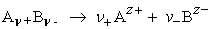be the chemical equation for the dissolution of the strong electrolyte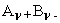with , ν+ν- ,i.e. an asymmetrical electrolyte, and v = ν+ + ν-.

The solute activity a is defined as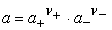(S1)

The mean activity a± is defined as the geometrical mean of the separate ion activities: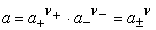or: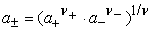(S2)

Substituting a+ = γ+m+ and a- = γ- m- in (S2) leads to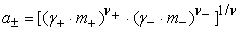(S3)

Because m+ = ν + m and m- = ν- m we can write: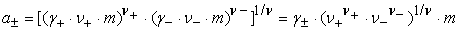(S4)

with a definition of the mean activity coefficient analogous to the mean activity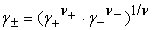(S5)

and a factor W characteristic for the electrolyte in question: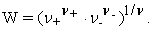Thus equation 4, formulated shortly: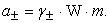(S6)

Substitution of equations (S2) and (S6) in the Nernst equation gives the following result: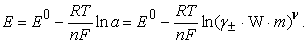(S7)

With molality as a measure of composition and results of scientific equilibrium measurements, we have an ultimate check if the Nernst equation is an empirical law and the table of standard electrode potentials contains empirical values.

Therefore we consider γ± to be known for the electrolyte in question from literature. When in (S7) the power ν is placed before the logarithm and the ln term is split up in a constant part E0′ with E0, γ± , ν/n and W, and a m dependent part h log m, the following equation results: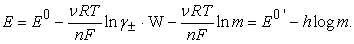(S8)This work is licensed under a Creative Commons Attribution 4.0 International License. To view a copy of this license, visit http://creativecommons.org/licenses/by/4.0/